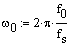## Removing 60Hz from ECG using digital band stop filterLet’s make a filter, which filters off the 60Hz frequency from the ECG signal. As we know, the American power supply is 60Hz. This is a common noise in biomedical signals while the industrial power supply powers them. This type of noise can be defined easily and can be filtered as parameters of noise are known.Here is one example of how to implement an FIR filter using mathematical tools, like Matlab. This can be done using a microcontroller, like ARM or even AVR, because the frequencies are up to 1 kHz.Initial conditions: f0=60Hz – power supply frequency;fs=500Hz – sampling rate;frequencies who define complex zeros: we get w0=0.754;Positions of complex zeros: Zeros and poles in z plane System Function From it we can calculate filter coefficients: And filter coefficients: b3 = 1; b2 = -2cos(w0); b1 = 1 Also we know that: And here we get filter characteristics: We have a band stop filter at 60Hz, and its jam at 60Hz is -300dB. Bellow is a filter structure: Now using this filter we can filter ECG signal: As you can see, this is a simple FIR filter. In other words, there is nothing more than an average function that doesn’t need…